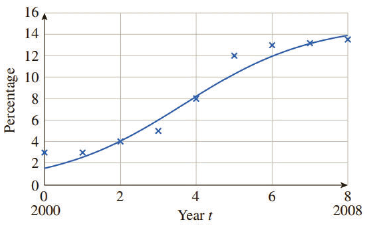Chapter 11.5, Problem 103EFinite Mathematics and Applied Cal...

7th Edition
Stefan Waner + 1 other
ISBN: 9781337274203

Solutions

Chapter
SectionFinite Mathematics and Applied Cal...

7th Edition
Stefan Waner + 1 other
ISBN: 9781337274203
Textbook Problem

Subprime Mortgages during the Housing Bubble During the real estate run-up in 2000–2008 the percentage of mortgages issued in the United States that were subprime (normally classified as risky) could be approximated by A ( t ) = 15.0 1 + 8.6 e − 0.59 t  percent ( 0 ≤ t ≤ 8 ) t years after the start of 2000.55Subprime mortgagesHow fast, to the nearest 0.1%, was the percentage increasing at the start of 2003? How would you check that the answer is approximately correct by looking at the graph? [HINT: See Example 3.]

To determine

To calculate: The increase of mortgages at the start of 2003 if the percentage of mortgages issued that were risky is given by the equation A(t)=151+8.6e0.59t percent (0t8) t years after the start of 2000 and to check if the answer is correct with the help of the given graph.

Explanation

Given Information:

The percentage of mortgages issued that were risky is given by the equation A(t)=151+8.6e0.59t percent (0t8) t years after the start of 2000.

The graph is as follows:

Formula used:

The following derivative formula is used:

ddx(ex)=ex

Calculation:

Consider that percentage of mortgages issued that were risky is given by the equation A(t)=151+8.6e0.59t percent (0t8) t years after the start of 2000.

Re-write A(t)=151+8.6e0.59t percent as A(t)=15(1+8.6e0.59t)1

The rate of change of A(t) is equal to the rate of change of the percentage.

Still sussing out bartleby?

Check out a sample textbook solution.

See a sample solution

The Solution to Your Study Problems

Bartleby provides explanations to thousands of textbook problems written by our experts, many with advanced degrees!

Get Started

Find more solutions based on key concepts